Courses

# Maxwell’s Equations: Electromagnetic Waves Predicted and Observed Physics Notes | EduRev

## Physics : Maxwell’s Equations: Electromagnetic Waves Predicted and Observed Physics Notes | EduRev

The document Maxwell’s Equations: Electromagnetic Waves Predicted and Observed Physics Notes | EduRev is a part of the Physics Course Basic Physics for IIT JAM.
All you need of Physics at this link: Physics

The Scotsman James Clerk Maxwell (1831–1879) is regarded as the greatest theoretical physicist of the 19th century. Although he died young, Maxwell not only formulated a complete electromagnetic theory, represented by Maxwell’s equations, he also developed the kinetic theory of gases and made significant contributions to the understanding of color vision and the nature of Saturn’s rings.
James Clerk Maxwell, a 19th-century physicist, developed a theory that explained the relationship between electricity and magnetism and correctly predicted that visible light is caused by electromagnetic waves. (credit: G. J. Stodart)
This black and white engraving shows physicist James Clerk Maxwell as a Victorian era gentleman dressed in bowtie, vest, and jacket, and sporting a full, graying beard and moustache.
Maxwell brought together all the work that had been done by brilliant physicists such as Oersted, Coulomb, Gauss, and Faraday, and added his own insights to develop the overarching theory of electromagnetism. Maxwell’s equations are paraphrased here in words because their mathematical statement is beyond the level of this text. However, the equations illustrate how apparently simple mathematical statements can elegantly unite and express a multitude of concepts—why mathematics is the language of science.
Maxwell’s Equations

1. Electric field lines originate on positive charges and terminate on negative charges. The electric field is defined as the force per unit charge on a test charge, and the strength of the force is related to the electric constant ε0, also known as the permittivity of free space. From Maxwell’s first equation we obtain a special form of Coulomb’s law known as Gauss’s law for electricity.
2. Magnetic field lines are continuous, having no beginning or end. No magnetic monopoles are known to exist. The strength of the magnetic force is related to the magnetic constant μ0, also known as the permeability of free space. This second of Maxwell’s equations is known as Gauss’s law for magnetism.
3. A changing magnetic field induces an electromotive force (emf) and, hence, an electric field. The direction of the emf opposes the change. This third of Maxwell’s equations is Faraday’s law of induction, and includes Lenz’s law.
4. Magnetic fields are generated by moving charges or by changing electric fields. This fourth of Maxwell’s equations encompasses Ampere’s law and adds another source of magnetism—changing electric fields.

Maxwell’s equations encompass the major laws of electricity and magnetism. What is not so apparent is the symmetry that Maxwell introduced in his mathematical framework. Especially important is his addition of the hypothesis that changing electric fields create magnetic fields. This is exactly analogous (and symmetric) to Faraday’s law of induction and had been suspected for some time, but fits beautifully into Maxwell’s equations.
Symmetry is apparent in nature in a wide range of situations. In contemporary research, symmetry plays a major part in the search for sub-atomic particles using massive multinational particle accelerators such as the new Large Hadron Collider at CERN.
Making Connections: Unification of Forces
Maxwell’s complete and symmetric theory showed that electric and magnetic forces are not separate, but different manifestations of the same thing—the electromagnetic force. This classical unification of forces is one motivation for current attempts to unify the four basic forces in nature—the gravitational, electrical, strong, and weak nuclear forces.
Since changing electric fields create relatively weak magnetic fields, they could not be easily detected at the time of Maxwell’s hypothesis. Maxwell realized, however, that oscillating charges, like those in AC circuits, produce changing electric fields. He predicted that these changing fields would propagate from the source like waves generated on a lake by a jumping fish.
The waves predicted by Maxwell would consist of oscillating electric and magnetic fields—defined to be an electromagnetic wave (EM wave). Electromagnetic waves would be capable of exerting forces on charges great distances from their source, and they might thus be detectable. Maxwell calculated that electromagnetic waves would propagate at a speed given by the equation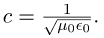When the values for μ0 and ε0 are entered into the equation for c, we find that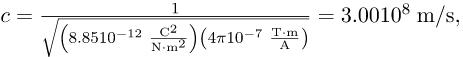which is the speed of light. In fact, Maxwell concluded that light is an electromagnetic wave having such wavelengths that it can be detected by the eye.
Other wavelengths should exist—it remained to be seen if they did. If so, Maxwell’s theory and remarkable predictions would be verified, the greatest triumph of physics since Newton. Experimental verification came within a few years, but not before Maxwell’s death.
Hertz’s Observations
The German physicist Heinrich Hertz (1857–1894) was the first to generate and detect certain types of electromagnetic waves in the laboratory. Starting in 1887, he performed a series of experiments that not only confirmed the existence of electromagnetic waves, but also verified that they travel at the speed of light.
Hertz used an AC RLC (resistor-inductor-capacitor) circuit that resonates at a known frequency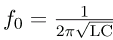and connected it to a loop of wire as shown in. High voltages induced across the gap in the loop produced sparks that were visible evidence of the current in the circuit and that helped generate electromagnetic waves.
Across the laboratory, Hertz had another loop attached to another RLC circuit, which could be tuned (as the dial on a radio) to the same resonant frequency as the first and could, thus, be made to receive electromagnetic waves. This loop also had a gap across which sparks were generated, giving solid evidence that electromagnetic waves had been received.
The apparatus used by Hertz in 1887 to generate and detect electromagnetic waves. An RLC circuit connected to the first loop caused sparks across a gap in the wire loop and generated electromagnetic waves. Sparks across a gap in the second loop located across the laboratory gave evidence that the waves had been received.
The circuit diagram shows a simple circuit containing an alternating voltage source, a resistor R, capacitor C and a transformer, which provides the impedance. The transformer is shown to consist of two coils separated by a core. In parallel with the transformer is connected a wire loop labeled as Loop one Transmitter with a small gap that creates sparks across the gap. The sparks create electromagnetic waves, which are transmitted through the air to a similar loop next to it labeled as Loop two Receiver. These waves induce sparks in Loop two, and are detected by the tuner shown as a rectangular box connected to it.
Hertz also studied the reflection, refraction, and interference patterns of the electromagnetic waves he generated, verifying their wave character. He was able to determine wavelength from the interference patterns, and knowing their frequency, he could calculate the propagation speed using the equation υ = fλ (velocity—or speed—equals frequency times wavelength). Hertz was thus able to prove that electromagnetic waves travel at the speed of light. The SI unit for frequency, the hertz (1Hz = 1 cycle/ sec), is named in his honor.
Section Summary

• Electromagnetic waves consist of oscillating electric and magnetic fields and propagate at the speed of light c. They were predicted by Maxwell, who also showed that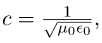where μ0 is the permeability of free space and  and ε0 is the permittivity of free space.
• Maxwell’s prediction of electromagnetic waves resulted from his formulation of a complete and symmetric theory of electricity and magnetism, known as Maxwell’s equations.
• These four equations are paraphrased in this text, rather than presented numerically, and encompass the major laws of electricity and magnetism. First is Gauss’s law for electricity, second is Gauss’s law for magnetism, third is Faraday’s law of induction, including Lenz’s law, and fourth is Ampere’s law in a symmetric formulation that adds another source of magnetism—changing electric fields.

Problems & Exercises
Verify that the correct value for the speed of light c is obtained when numerical values for the permeability and permittivity of free space (μ0 and ε0) are entered into the equation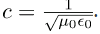Show that, when SI units for μ0 and ε0 are entered, the units given by the right-hand side of the equation in the problem above are m/s.

Offer running on EduRev: Apply code STAYHOME200 to get INR 200 off on our premium plan EduRev Infinity!

,

,

,

,

,

,

,

,

,

,

,

,

,

,

,

,

,

,

,

,

,

;